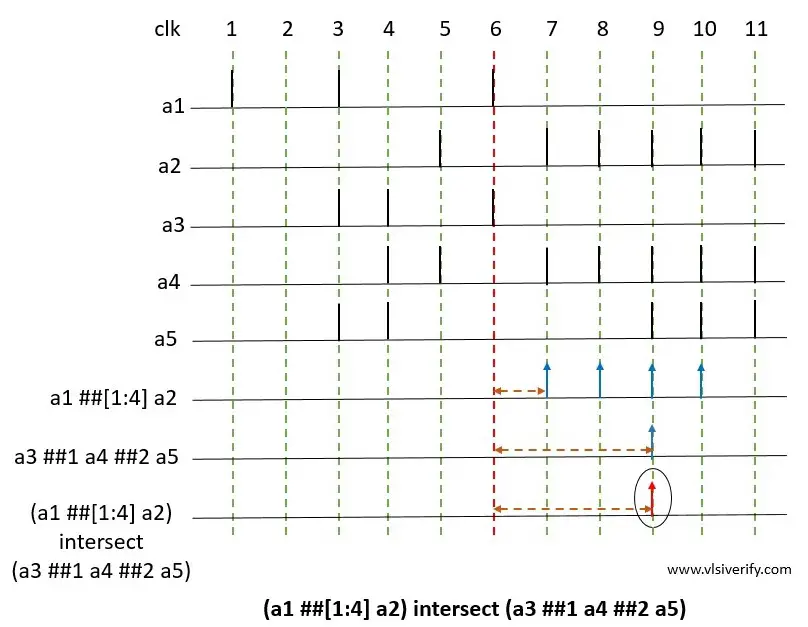Tutorials

The binary intersect operator is used when two sequence operands are expected to match, and the end time of both operand sequences must be the same.

Syntax:

``<seq_exp> intersect <seq_exp>``

The requirement for intersect operation match

1. Both operand sequence lengths must be the same.
2. Both operands must match. In case one operand matches, it waits for another operand to match.
3. The end time of the resultant or composite sequence is equal to the end time of the matched operand sequences.

## Example for Intersection of two sequences

``(a1 ##[1:4] a2) intersect (a3 ##1 a4 ##2 a5)``### Explanation

a1 ##[1:4] a2: The first operand a1 evaluates to true followed by a2 which evaluates to true for 1,2,3, or 4 clock ticks later. So, sequence matches at clock ticks 7,8,9, and 10 respectively.

a3 ##1 a4 ##2 a5: The operand a3 evaluates to true followed by a4 one clock tick later, followed by a5 two clock ticks later i.e. at clock tick 9.

(a1 ##1 a2) intersect (a3 ##1 a4 ##2 a5): The resultant sequence is matched at clock tick 9 as both of individual operand sequence has matched.

SystemVerilog Assertions